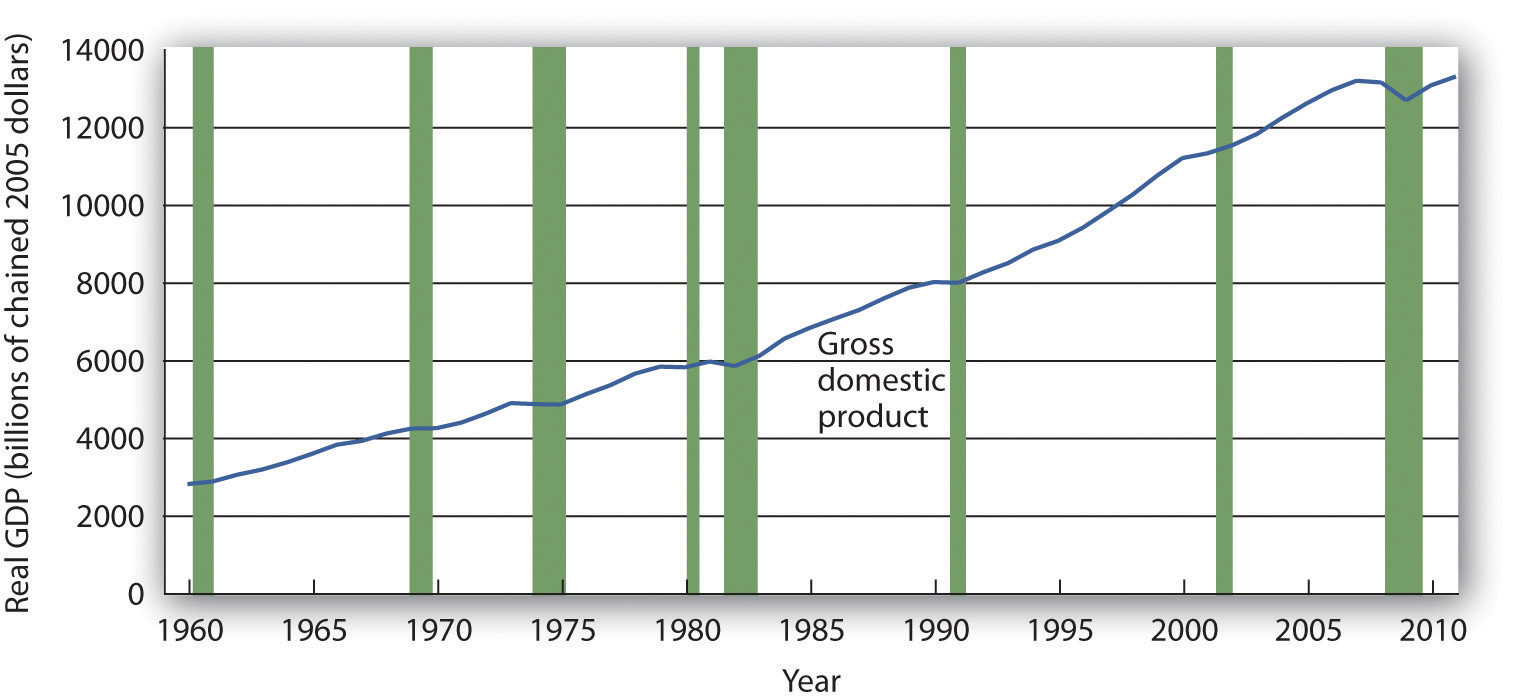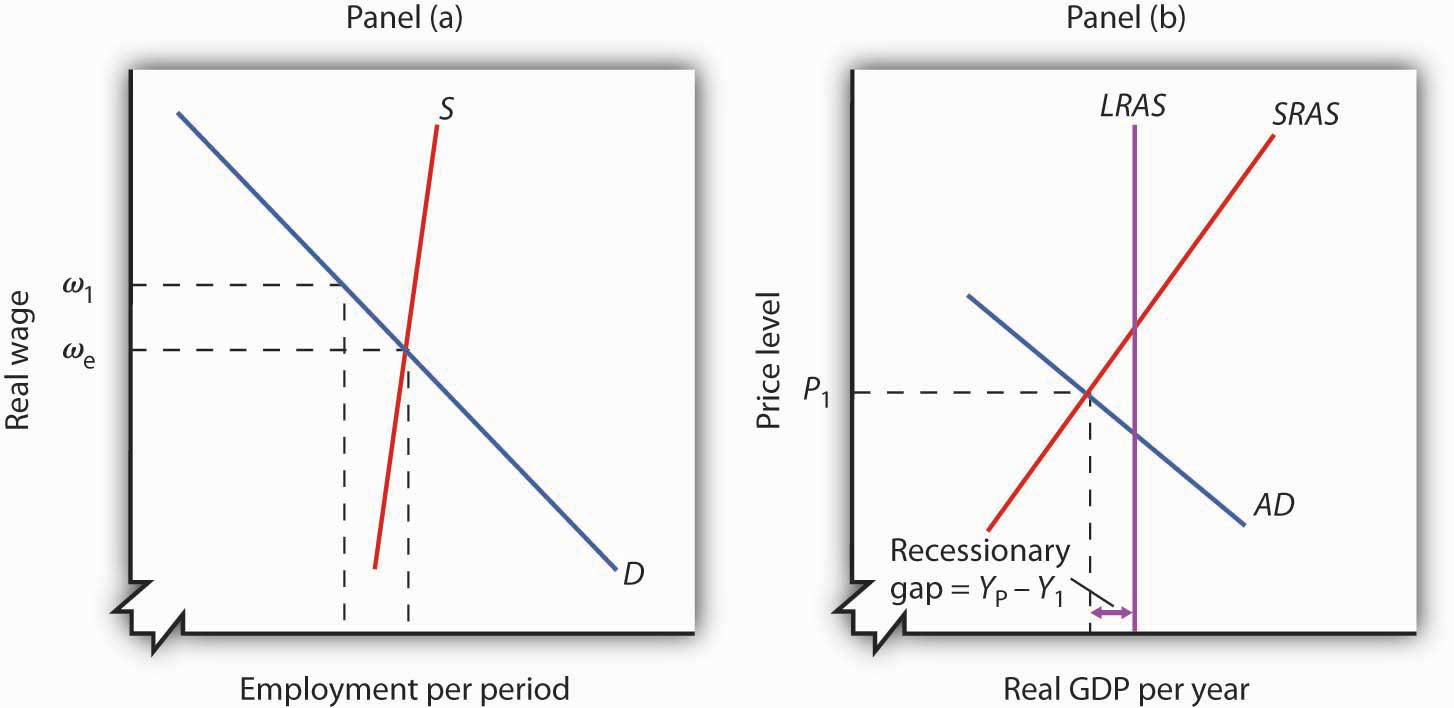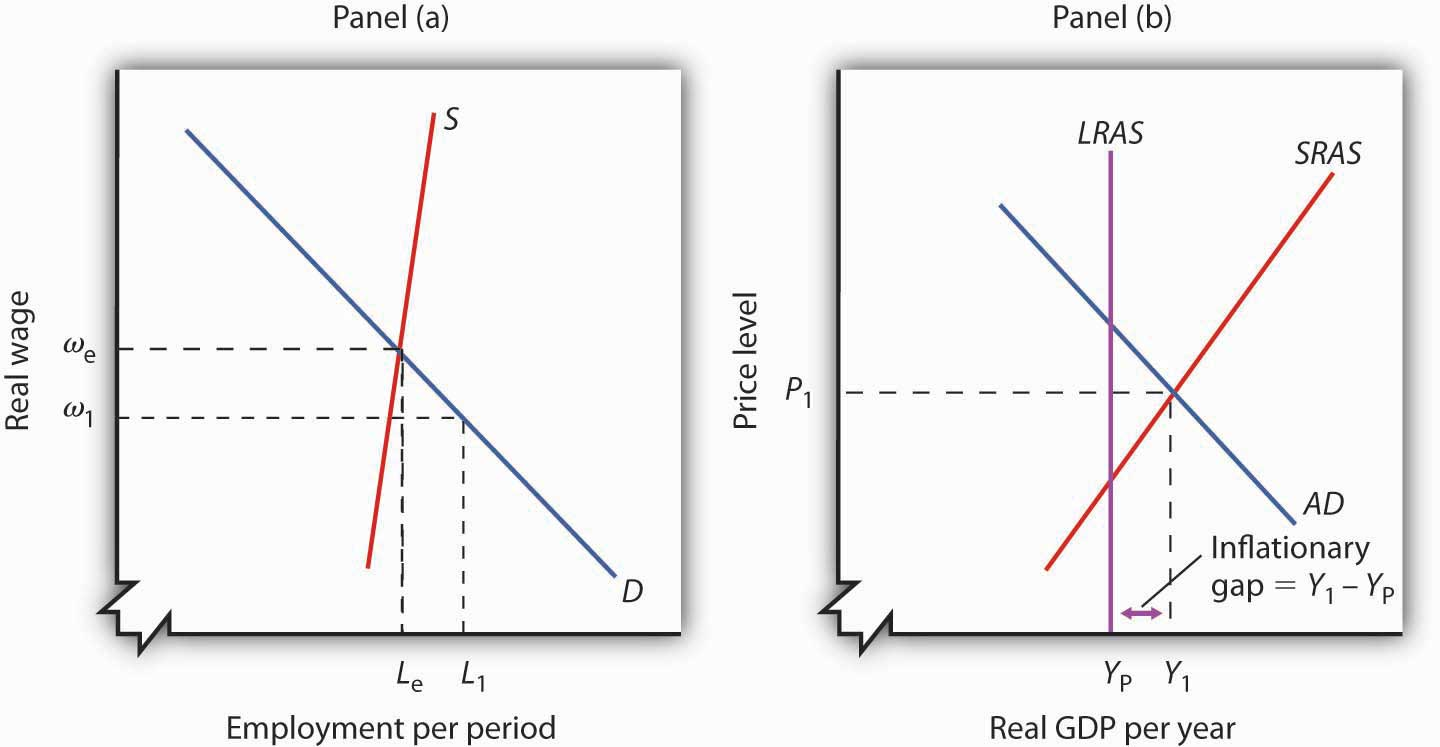Unit 4 Study Guide and Review: Aggregate Economic Activities and Fluctuations

4a: Graphically represent and interpret a short-run aggregate supply curve, and explain why it slopes upward and factors leading to its shift outward or inward.

• Define the product supply curve and explain why it slopes upward.

The short-run aggregate supply curve resembles the product supply curve we reviewed in Unit 1. The short-run aggregate supply curve slopes upward to reflect the positive relationship between price level (on the vertical axis) and real GDP (on the horizontal axis).

Just as when we studied the supply and demand curves for a limited customer base or business, remember that the short-run aggregate supply curve is a ratio plotted on a graph that depicts the relationship between price (the y axis) and Real GDP (the x axis), so a price fluctuation will cause a movement along the short-run aggregate supply curve (you can see the points on the supply curve refer to Real GDP based on a given price). Price fluctuations do not shift the short-run aggregate supply curve.

Factors that shift the short-run aggregate supply curve include:

• Aggregate stock, or amount, of available capital;
• Aggregate stock, or amount, of available natural resources;
• Aggregate level of technology and innovation; and,
• Aggregate cost, or prices of the factors of production.

Review the short-run aggregate supply curve in the following resources.

4b: Define aggregate demand, and identify the reasons for its negative slope.

• Define the product demand curve and explain why it slopes downward.

The aggregate demand curve resembles the product demand curve we reviewed in Unit 1. The aggregate demand curve slopes downward to reflect the negative relationship between price level (on the vertical axis) and real GDP (on the horizontal axis).

• Define the wealth effectinterest rate effect, and international trade effect.
• Why does a change in the price level lead to a change in demand in terms of real GDP?

Three important effects that lead to the negative slope of the aggregate demand curve, which are all caused by a change in price level, include: the wealth effect, the interest rate effect, and the international trade effect.

• Define consumptioninvestmentgovernment spending, and net exports and explain why changes in these variables cause the aggregate demand curve to shift.
• Define the spending multiplier.
• What can we say about the total effect on aggregate demand on Real GDP, considering the role of the spending multiplier?

Just as when we studied the supply and demand curves for a limited customer base or business, the aggregate demand curve is a ratio plotted on a graph that depicts the relationship between price level (the y axis) and Real GDP (the x axis) from a national perspective: a price level fluctuation will cause a movement along the aggregate demand curve (you can see the points on the aggregate demand curve refer to Real GDP based on a given price level). Price level fluctuations do not shift the aggregate demand supply curve.

Factors that shift the aggregate demand curve include national levels of: consumption, investment, government spending. and net exports.

Review the aggregate demand curve in the following resources.

4c: Explain the factors leading to a shift in the consumption function.

• Define marginal propensity to consume (MPC).
• How does MPC relate to the slope of the consumption function?
• Define marginal propensity to save (MPS) and explain how it is related to MPC.
• What variables shift the consumption function?
• What is the relationship between consumption and aggregate expenditures in the economy?

To analyze the consumption function, it is important to determine the relationship between disposable income and the choice households make to spend or save.

Review the consumption function in the following resources.

4d: Define short-run equilibrium and long-run equilibrium, and discuss how they differ.

Review short run and long run equilibrium in learning outcome 4c above.

4e: Describe the four phases of a business cycle, including references to income and real output.

• Define economic contractionrecession, and trough.
• Define economic expansionboom, and peak.

Business cycles describe the periodic natural fluctuations in the economy, in which recession, economic expansion, and economic booms alternate. The phases of a business cycle include peak, contraction, recession, trough, recovery, and expansion.

Review Figure 5.2 which depicts the Great Recession the United States experienced from 2008 to 2009, and an economic expansion from 2009 to 2011.

Figure 5.2 Expansions and Recessions, 1960–2011The chart shows movements in real GDP since 1960. Recessions—periods of falling real GDP—are shown as shaded areas. On average, the annual rate of growth of real GDP over the period was 3.1 percent per year.

Growth of Real GDP and Business Cycles, Principles of Macroeconomics.

Review the business cycle in the following resources.

4f: Graphically represent and interpret a long-run aggregate supply curve, and explain its connection to natural level of unemployment.

• Define the natural level of employment.
• What types of employment does the natural level of employment represent and exclude?
• What is the shape of the long-run aggregate supply (LRAS) curve?
• What factors shift the aggregate supply curve over time?

The long-run aggregate supply curve compares the price level to the level of real GDP in the long-run. Note that we draw the long-run aggregate supply curve vertically at a fixed level of real GDP (the level of real GDP associated with the "natural level of employment"). An economy that experiences employment levels lower than the "natural level of employment," indicates the presence of cyclical unemployment.

Review the aggregate supply curve in Aggregate Demand and Aggregate Supply from Principles of Macroeconomics, and the video Long-Run Aggregate Supply from Khan Academy.

4g: Describe how short-run equilibriums occur above and below the output level associated with the natural rate of unemployment.

• Define recessionary gap.
• What can we say about the level of real GDP during a recessionary gap?
• What is the level of employment during a recessionary gap?
• Do sticky wages cause a recessionary gap to persist?
• What actions can a government take to help re-establish the long-run equilibrium to eliminate a recessionary gap?

The intersection of the short-run aggregate demand and supply curves describes a short-run equilibrium of the price level and real GDP. We can describe this equilibrium as a long-run equilibrium, when the level of real GDP equals the level associated with the "natural level of employment," or equals the real GDP for the long-run aggregate supply (LRAS) curve.

A Recessionary Gap

A recessionary gap is not the same as a recession. A recessionary gap describes an economic situation where unemployment exceeds the natural levels. A recession, on the other hand, indicates a falling real GDP. A recession can, and often does, lead to a recessionary gap.

Figure 7.10 A Recessionary GapIf employment is below the natural level, as shown in Panel (a), then output must be below potential. Panel (b) shows the recessionary gap YP − Y1, which occurs when the aggregate demand curve (AD) and the short-run aggregate supply curve (SRAS) intersect to the left of the long-run aggregate supply curve (LRAS).

Aggregate Demand and Aggregate Supply, Principles of Macroeconomics.

Review the recessionary gap in Aggregate Demand and Aggregate Supply from Principles of Macroeconomics.

• Define inflationary gap.
• What can we say about the level of real GDP during an inflationary gap?
• What is the level of employment during an inflationary gap?
• Do sticky wages cause an inflationary gap to persist?
• What actions can help reestablish the long-run equilibrium to eliminate an inflationary gap?

An Inflationary Gap

An inflationary gap is a long-run disequilibrium situation. An inflationary gap describes the opposite situation to a recessionary graph, as illustrated in the graphs.

Figure 7.11 An Inflationary GapPanel (a) shows that if employment is above the natural level, then output must be above potential. The inflationary gap, shown in Panel (b), equals Y1 − YP. The aggregate demand curve (AD) and the short-run aggregate supply curve (SRAS) intersect to the right of the long-run aggregate supply curve (LRAS).

Aggregate Demand and Aggregate Supply, Principles of Macroeconomics.

Review the inflationary gap in Aggregate Demand and Aggregate Supply from Principles of Macroeconomics.

• Without active government policy, how will adjustments among wages and prices (unsticking) eventually lead to a shift of the short-run aggregate supply curve toward the long-run equilibrium real GDP?
• Explain what classical economic theorists and Keynesians recommend policy makers do when a long-run disequilibrium occurs, to help the economy move toward equilibrium?

Restoring Long-Run Macroeconomic Equilibrium

A common controversy exists among the options a country has to address long-run disequilibrium. In the classical view, an economy can recover from a downturn through adjustments in the labor market. This requires flexible wages and prices.

We know wages and prices are sticky. A long-run disequilibrium can persist until the wages and prices are able to adjust to changes in the economy. Wages and prices need to unstick.

Review classical and Keynesian views regarding how quickly an economy self-adjusts in the following resources.

Unit 4 Vocabulary

• Aggregate demand/aggregate supply model
• Aggregate expenditures
• Aggregate supply (AS)
• Aggregate supply (AS) curve
• Consumption
• Depression
• Economic boom
• Economic contraction
• Economic expansion
• Economic recession
• Full-employment GDP
• Government spending
• Inflationary gap
• Interest rate effect
• Investment
• Long run aggregate supply (LRAS) curve
• Marginal propensity to consume
• Marginal Propensity to save
• Natural level of employment
• Neoclassical economists
• Net exports
• Peak
• Potential GDP
• Product supply curve
• Production possibility curve
• Recession
• Recessionary gap
• Short run aggregate supply (SRAS) curve
• Spending multiplier
• Stagflation
• Sticky prices
• Sticky wages
• Trough
• Wealth effect